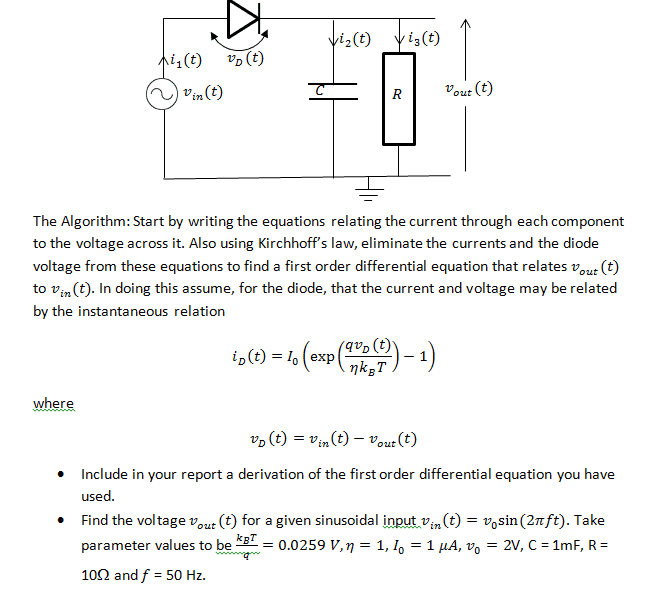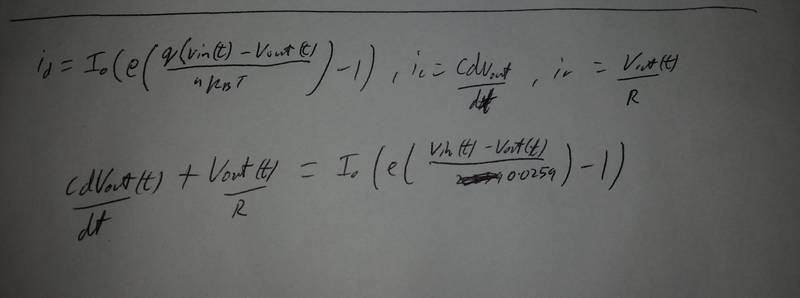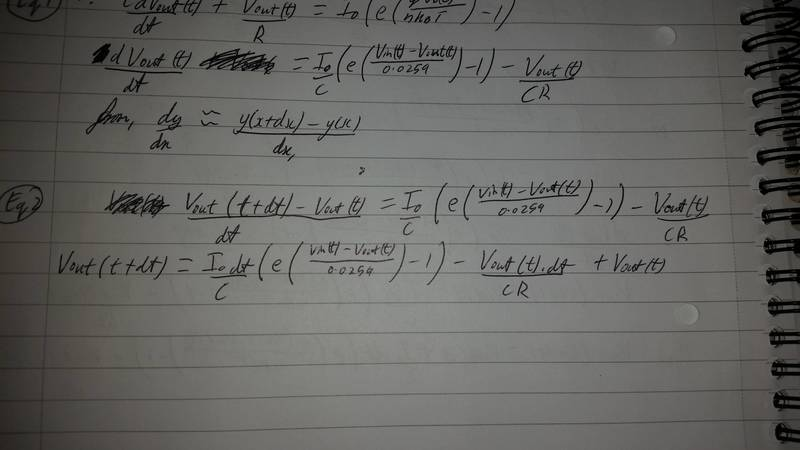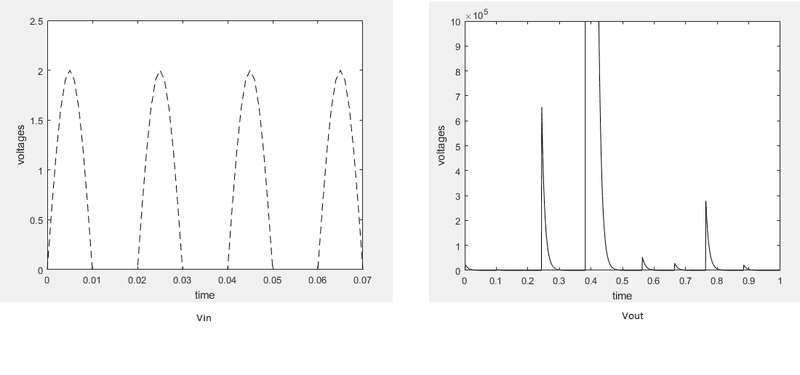# Diode - RC circuit differential equation

• Engineering

## Homework StatementI'm having difficulty deriving the differential equation, this is what I have so far. In order to solve it, I will be using Matlab, and I'll be using the equation dy/dx ≈ (y(x + dx) - y(x))/dx. Is my derivation correct so far?

In picture.

## The Attempt at a SolutionIf it's correct, do I just need to make DVout/dt the subject, then apply the approx. derivative equation to then calculate the values?

Last edited by a moderator:

gneill
Mentor
You're on the right path, but ffter you've made your substitution for the derivative you'll want to isolate the "new" value ##v_{out}(t + dt)## so that you can calculate the new ##v_{out}## from its current value over the timestep dt.

You're on the right path, but ffter you've made your substitution for the derivative you'll want to isolate the "new" value ##v_{out}(t + dt)## so that you can calculate the new ##v_{out}## from its current value over the timestep dt.
I've updated it as follows:The problem I've had with this is that when I write a MATLAB program to solve it, and display it as a graph, it definitely doesn't seem correct. For some reason, the voltages are incredibly small, i.e. 2x10^-16.

#### Attachments

Last edited by a moderator:
gneill
Mentor
Your final expression for ##v_{out}(t + dt)## looks fine. Must be something to do with how you are doing the integration in MATLAB. So you'll be looking at debugging that. Maybe running for just a few timesteps and printing out the various variable values will help.

I'm not very familiar with MATLAB, but I get reasonable results using the same expression in MathCad.

Your final expression for ##v_{out}(t + dt)## looks fine. Must be something to do with how you are doing the integration in MATLAB. So you'll be looking at debugging that. Maybe running for just a few timesteps and printing out the various variable values will help.

I'm not very familiar with MATLAB, but I get reasonable results using the same expression in MathCad.
If I post the code, would you be able to see where I'm going wrong if possible? The MATLAB language is rather basic, or at least what I've wrote.

gneill
Mentor
You can post the code (be sure to use code ... \code tags around it to preserve formatting). But you really should get some experience with debugging your own code.

You can post the code (be sure to use code ... \code tags around it to preserve formatting). But you really should get some experience with debugging your own code.
I agree, I have tried debugging it although I honestly can't see what's wrong.

Code:
%Variables and constants
C = 0.001;
R = 10;
V0 = 2;
f = 50;
I = 0.000001;
dt = 0.01;
t = 0:dt:2;

Vin = V0 * sin(2*pi*f*t); %Input voltage
Vout(1) = 0; %Initial Vout

for n = 1:length(Vin) - 1,
Vout(n + 1) = Vout(n) - ((Vout(n) * dt) / (R * C)) + ((I * dt / C) * (exp((Vin(n) - Vout(n) ) / 0.0259) - 1));
end

plot(t, Vin, 'k--', t, Vout, 'k')
ylim([0 2]); %Limits y-axis
xlabel('time');
ylabel('voltages');

gneill
Mentor
Check your timestep size. How does it compare to the period of the input waveform? What's a reasonable total time to cover, say, two full periods?

Check your timestep size. How does it compare to the period of the input waveform? What's a reasonable total time to cover, say, two full periods?
I've now tidied it up, but the graph doesn't look promising. The Vin graph is fine, but the Vout isn't good at all, Vout does get quite large.
This is the new code:
Code:
%Variables and constants
C = 0.001;
R = 10;
V0 = 2;
f = 50;
I = 0.000001;
dt = 0.001;
t = 0:dt:0.08;

Vin = V0 * sin(2*pi*f*t); %Input voltage
Vout(1) = 0; %Initial Vout

for n = 1:length(Vin) - 1,
Vout(n + 1) = Vout(n) - ((Vout(n) * dt) / (R * C)) + ((I * dt / C) * (exp((Vin(n) - Vout(n) ) / 0.0259) - 1));
end

plot(t, Vin, 'k--', t, Vout, 'k')
ylim([0 2.5]); %Limits y-axis
xlabel('time');
ylabel('voltages')

Here are the graphs:gneill
Mentor
Vin should be between + and - 2 Volts, so you'll want to adjust the y-axis for its plot.

I think that your dt should be even smaller to begin with. Try something like a hundred "samples" per cycle of the input.

I see that your output voltage scale looks rather large. You'll need to find out how that happened. Try printing out the terms of the equation for a few iterations and see where they're headed.

Vin should be between + and - 2 Volts, so you'll want to adjust the y-axis for its plot.

I think that your dt should be even smaller to begin with. Try something like a hundred "samples" per cycle of the input.

I see that your output voltage scale looks rather large. You'll need to find out how that happened. Try printing out the terms of the equation for a few iterations and see where they're headed.
Thank you very much, it is working perfectly now. I manually adjusted the scale for Vout since it was very much of the graph. Once again, thank you for your help.

gneill
Mentor
Glad I could help